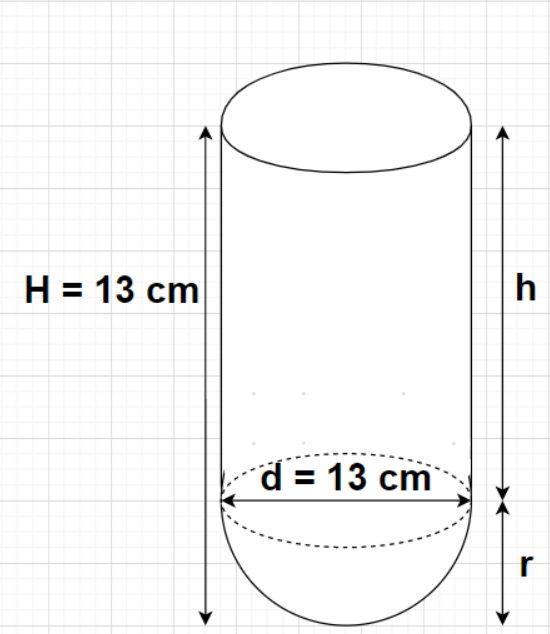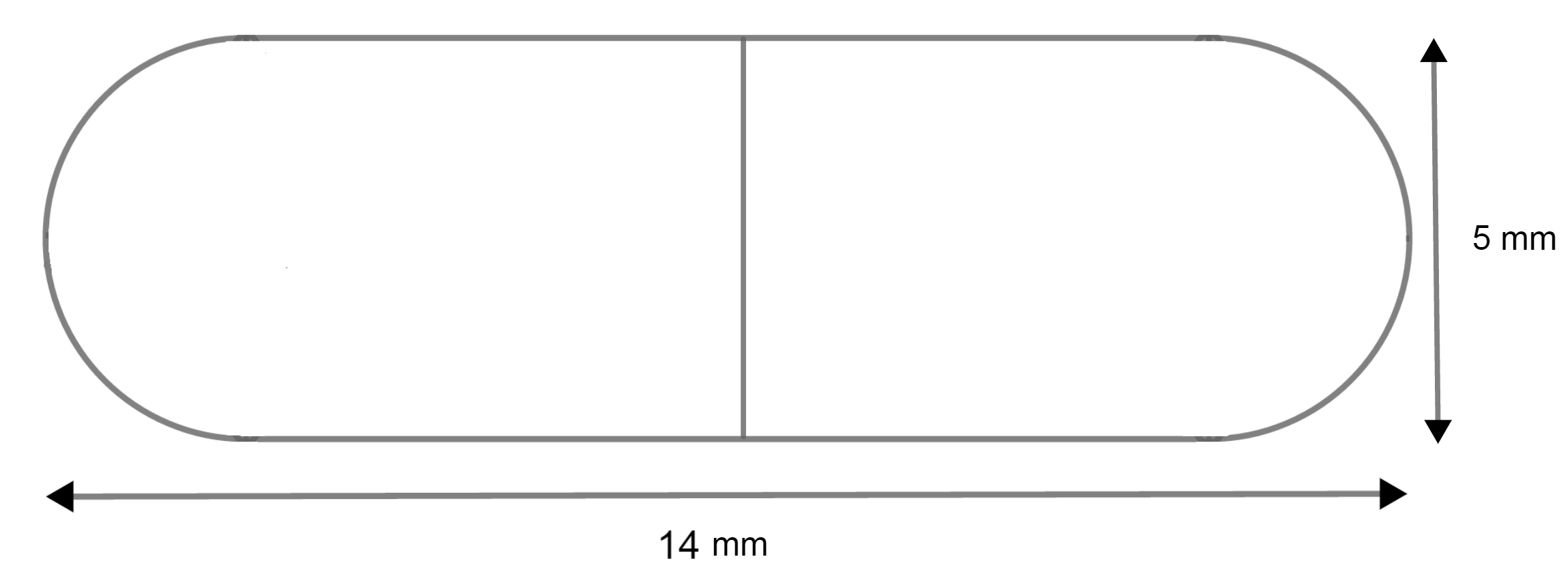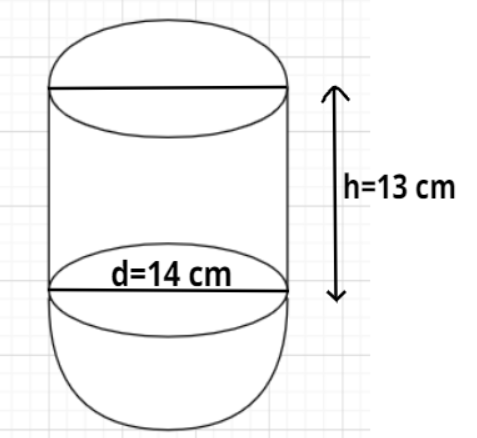Filters
Latest Questions
Mathematics
Surface area of a combination of solids
A vessel is in the form of a hemispherical bowl surmounted by a hollow cylinder of same diameter. The diameter of the hemispherical bowl is 14 cm and the total height of the vessel is 13 cm. Find the total surface area of the vessel. (Use $\pi = \dfrac{{22}}{7}$)Mathematics
Surface area of a combination of solids
A medicine capsule is in the shape of a cylinder with two hemispheres stuck to each of its ends. The length of the entire capsule is 14mm and the diameter of the capsule is 5mm. Find its surface area.Mathematics
Surface area of a combination of solids
The length, breadth and height of a cuboid are 6 cm, 4 cm and 5 cm respectively. Find the total surface area of the cuboid.
Mathematics
Surface area of a combination of solids
A vessel in the form of a hollow hemisphere mounted by a hollow cylinder. The diameter of the hemisphere is 14 cm and the height of the cylindrical part is 13 cm. Find the inner surface area of the vessel.Mathematics
Surface area of a combination of solids
A hemispherical depression is cut out from one face of the cubical wooden block such that the diameter $l$ of the hemisphere is equal to the edge of the cube. Determine the surface area of the remaining solid.
Mathematics
Surface area of a combination of solids
From a solid cylinder whose height is 2.4 cm and diameter 1.4 cm, a conical cavity of the same height and same diameter is hollowed out. Find the total surface area of the remaining solid to the nearest cm2. (Use $\pi = \dfrac{{22}}{7}$)
Mathematics
Surface area of a combination of solids
A toy is in the form of a cone of radius3.5cm. Find the total surface area of the toy rounded off to the nearest integer.
Prev
1
Next